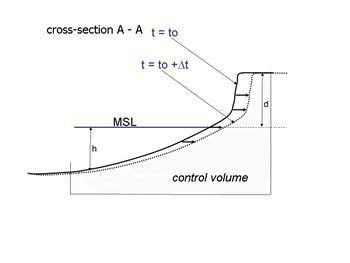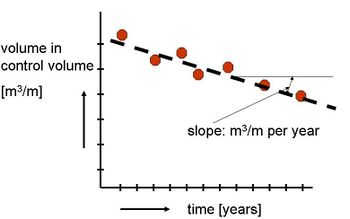# Example: Rate of recession versus volume rate of erosionFigure 1 Structural erosionFigure 2 Volume loss out of control volume due to structural erosion Rate of recession versus volume rate of erosion Figure 1 sketches a typical structural erosion case. Two cross-shore profiles have been indicated, representing the position of the profiles at two different moments within time. To a first approximation it can be assumed that the shape of the profiles are identical (the boundary conditions; e.g. wave climate and tides are the same indeed). The one profile can be found from the other by a horizontal shift. It is assumed that not only for example the waterline shifts in landward direction with a certain speed, but that this holds in fact for all depth contours. If on an average the waterline shows a recession of [math]r[/math] m/year, the annual loss of volume out of the control volume area [math]dV[/math] (m3/m.year) is approximately given by [math]r[/math] times [math](h + d)[/math]; see Figure 1 for the definition of [math]h[/math] and [math]d[/math]. The dune height [math]d[/math] with respect to MSL is easy to estimate. The under water part of the cross-shore profile [math]h[/math] which has to be taken into account, is more difficult to determine. Often the depth of the so-called active part of the profile is taken as representative. The closure depth (seaward boundary of the active part of the profile) is related to the annual wave climate. A first approximation for depth [math]h[/math] can be found by multiplying the significant wave height which is exceeded for one day a year, with 2 - 3. With actual profile measurements often a more accurate estimate of [math]h[/math] can be determined. Figure 2 gives the development of the volume [math]V[/math] of the control volume area with time of cross-shore profile. The slope [math]m = dV/dt[/math] (m3/m.year) is in this case a measure of the gravity of the erosion problem A similar figure like Figure 2 can be made of the recession of the position of the waterline (in m with respect to a reference point) with time of an eroding profile in a structural eroding part of the coast. The slope [math]r[/math] (m/year) is a measure for the erosion rate. From a morphological point of view representing erosion rates according to volumes is preferred above a representation according to distances. While the associated longshore sediment transports take place in the 'wet' part of the cross-shore profile, at the end of the day, also the mainland will permanently lose sediments. This can be understood by taking the erosion processes during a(n even moderate) storm into account. In a (seen over a number of years) stable condition the erosion of mainland is in fact only a temporary loss. In a structural erosion case this is (partly) a permanent loss. Sediments eroded from the mainland during the storm and settled at deeper water are eroded (by the gradient in the longshore sediment transport), before they have the chance and time to be transported back to the mainland. Although it looks like that the storm is the reason of the (permanent) erosion problem of the mainland, in this case the actual reason is the gradient in the longshore sediment transport (see Littoral drift and shoreline modelling). The storm is only to be considered as a necessary link in a chain of processes.